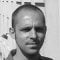Learn about power engineering and HV/MV/LV substations. Study specialized technical articles, electrical guides, and papers.

# Short review of resistance, reactance and impedance

Home / Technical Articles / Short review of resistance, reactance and impedance

## Resistance, R

Resistance is essentially friction against the motion of electrons. It is present in all conductors to some extent (except superconductors!), most notably in resistors. When alternating current goes through a resistance, a voltage drop is produced that is in-phase with the current.

Resistance is mathematically symbolized by the letter “R” and is measured in the unit of ohms (Ω).

## Reactance, X

Reactance is essentially inertia against the motion of electrons. It is present anywhere electric or magnetic ﬁelds are developed in proportion to applied voltage or current. respectively; but most notably in capacitors and inductors. When alternating current goes through a pure reactance, a voltage drop is produced that is 90° out of phase with the current.

Reactance is mathematically symbolized by the letter “X” and is measured in the unit of ohms (Ω).

## Impedance, Z

Impedance is a comprehensive expression of any and all forms of opposition to electron ﬂow, including both resistance and reactance. It is present in all circuits, and in all components. When alternating current goes through an impedance, a voltage drop is produced that is somewhere between 0” and 90° out of phase with the current.

Impedance is mathematically symbolized by the letter “Z” and is measured in the unit of ohms (Ω), in complex form.

Perfect resistors (Figure 1) possess resistance, but not reactance. Perfect inductors and perfect capacitors possess reactance, but not resistance.

All components possess impedance, and because of this universal quality, it makes sense to translate all component values (resistance, inductance, capacitance) into common terms of impedance as the ﬁrst step in analyzing an AC circuit.

The impedance phase angle for any component is the phase shill. between voltage across that component and current through that component. For a perfect resistor, the voltage drop and current are always in phase with each other, and so the impedance angle of a resistor is said to be . For an perfect inductor, voltage drop always leads current by 90°, and so an inductor’s impedance phase angle is said to be +90°.

For a perfect capacitor, voltage drop always lags current by 90°, and so a capacitor‘s impedance phase angle is said to be -90“.

Impedances in AC circuit behave analogously to resistances in DC circuit: they add in series, and they diminish in parallel. A revised version of Ohm’s Law, based on impedance rather than resistance, looks like this:

Ohm’s Law for AC circuit:

E = IZ   ;    I = E / Z   ;    Z = E / I

All quantities expressed in complex, not scalar form.

Kirchhoff’s Laws and all network analysis methods and theorems are true for AC circuits as well, so long as quantities are represented in complex rather than scalar form. While this qualiﬁed equivalence may be arithmetically challenging, it is conceptually simple and elegant. The only real difference between DC and AC circuit calculations is in regard to power.

Because reactance doesn’t dissipate power as resistance does, the concept of power in AC circuit is radically different from that of DC circuit.

Resource: Lessons in electric circuits, Volume II – AC, Sixth Edition

Get access to premium HV/MV/LV technical articles, electrical engineering guides, research studies and much more! It helps you to shape up your technical skills in your everyday life as an electrical engineer.### Edvard Csanyi

Electrical engineer, programmer and founder of EEP. Highly specialized for design of LV/MV switchgears and LV high power busbar trunking (<6300A) in power substations, commercial buildings and industry facilities. Professional in AutoCAD programming.

1.gowtham42
Jan 05, 2013

thank you very much sir today i learned a new thing about transformers thanks once again sir plz keep posting new articles every time so that no of students can enhance their knowledge in electrical engineering….

•Edvard
Jan 05, 2013

You’re welcome Gowtham42! Glad you like it!

I will continue publishing, that’s the promise ;)

2.lekhraj
Apr 08, 2012

HERE WE ARE TALKING ABOUT IMPENDANCE, WHICH IS FUNCTION OF AN ANGLE ……I HAVE LESS KNOLWEGE ABOUT THIS ANGLE LIKE ,HOW IT APPERS ACROOS CAPACITIVE LOAD OR WHY WE MASURE SUCH FACTS IN ANGLE……..